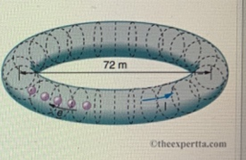# Problem: The Stanford Linear Accelerator Center (SLAC) operated on electron storage ring about 72.0 m in diameter called SPEAR (closed in 2009), in which a circulating beam of electrons moved at nearly the spend of light.Assuming that this electron beam had a current of I7 A, how many electrons were in the beam at any given movement?

###### FREE Expert Solution

The number of electrons can be calculated from the relation:

$\overline{){\mathbf{∆}}{\mathbf{Q}}{\mathbf{=}}{\mathbf{n}}{{\mathbf{q}}}_{{\mathbf{c}}}}$

The total amount of charge, ΔQ is obtained from the relation:

$\overline{){\mathbf{i}}{\mathbf{=}}\frac{\mathbf{∆}\mathbf{Q}}{\mathbf{∆}\mathbf{t}}}$

The relationship between speed, distance, and time is:

v = D/Δt

v = πd/Δt

95% (160 ratings)###### Problem Details

The Stanford Linear Accelerator Center (SLAC) operated on electron storage ring about 72.0 m in diameter called SPEAR (closed in 2009), in which a circulating beam of electrons moved at nearly the spend of light.Assuming that this electron beam had a current of I7 A, how many electrons were in the beam at any given movement?